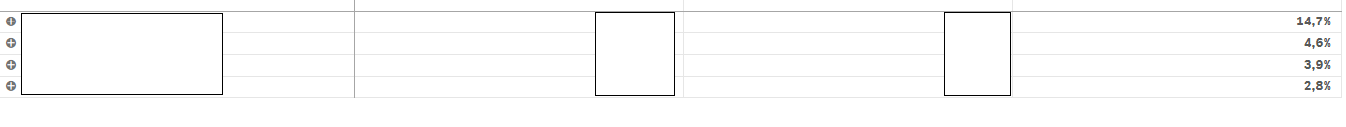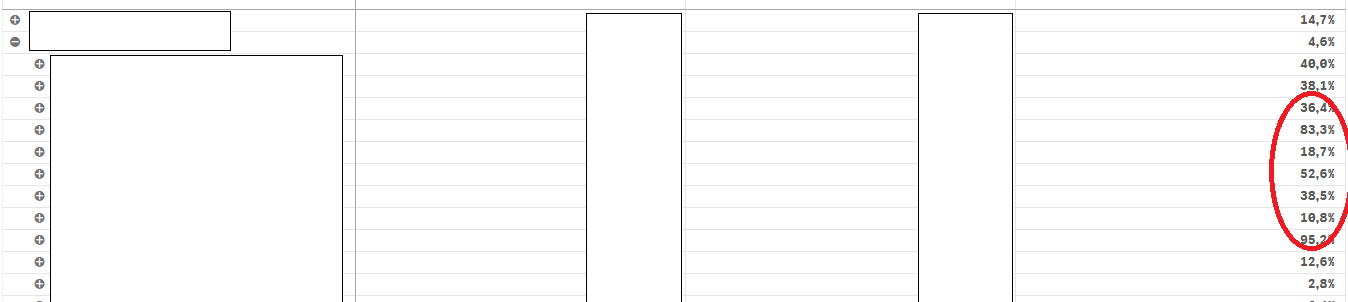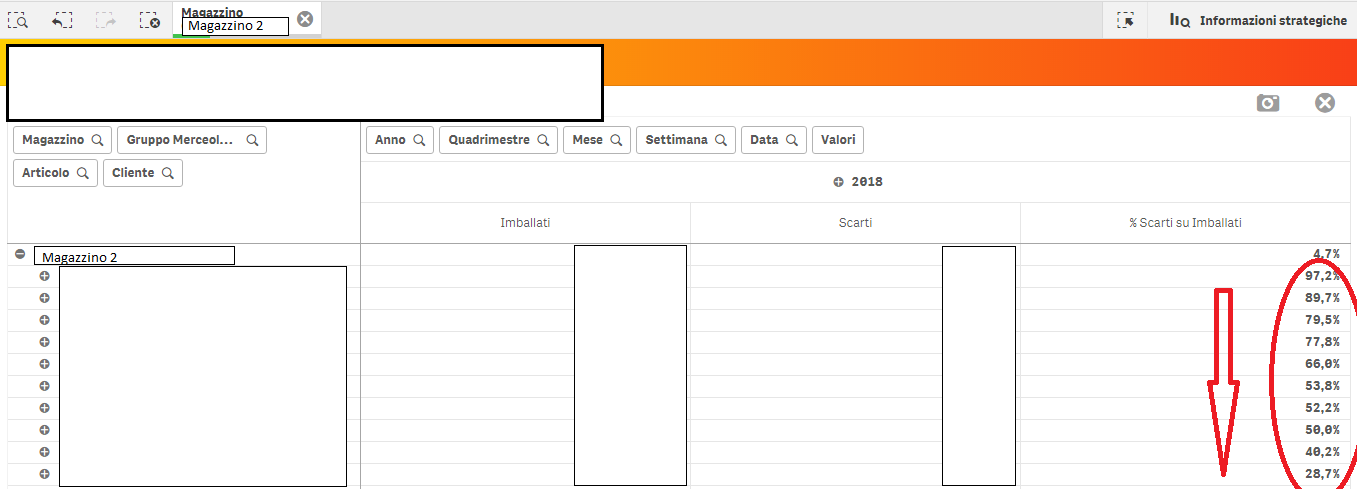# New to Qlik Sense

Discussion board where members can get started with Qlik Sense.

Highlighted
Contributor

## Problem to sort by measures in a pivot table

Good evening to all,

First of all sorry for my english.

I have a problem to sort data in a pivot table with four dimensions and two measures.

I have to sort value on one of the measures.

I set ‘sort by espression’ but it works only for the first dimension of the pivot, the external one.

I tried with different expression but nothig works.

I Actually use the function:

Aggr(Max(Aggr(Sum({<TipoOperazione = {'7'}, Causale = >}QuantitaFinale)/Sum({<TipoOperazione = {'7'}>} total QuantitaFinale),Articolo )),Articolo)

Where Articolo is the dimension and

Sum({<TipoOperazione = {'7'}, Causale = >}QuantitaFinale)/Sum({1}{<TipoOperazione = {'7'}>} total QuantitaFinale) is the measure

Do you have any suggestion?

Thanks in advice

Kind regards

19 Replies
Honored Contributor II

## Re: Problem to sort by measures in a pivot table

i think you just need to use your measure in sort by expression for all dimensions.

Contributor

## Re: Problem to sort by measures in a pivot table

Hi Andrea, thanks for reply, i have try this option but unfortunatly it does not work.

Use the measures: Sum({<TipoOperazione = {'7'}, Causale = >}QuantitaFinale)/Sum({1}{<TipoOperazione = {'7'}>} total QuantitaFinale) in the sort expression give the same result of the other expression i'm using.

Honored Contributor II

## Re: Problem to sort by measures in a pivot table

what kind of sorting are you looking for?

could you explain better what is your actual output and the expected one?

Contributor

## Re: Problem to sort by measures in a pivot table

What i want it's that all data are sorted in descending order for all the dimensions of the pivot table.

I set sort by espression for all the dimensions but it works only for the external dimension.

For the external dimension i have something like this.But when i expand the pivot the sorting does not work for the internal dimension and i have something like thisBy moving the internal dimensions outside the sort works correctly

Honored Contributor II

## Re: Problem to sort by measures in a pivot table

Hi Gloria,

which are dimensions and measures of your pivot table?

what's the expression you are using in desc sorting?

Contributor

## Re: Problem to sort by measures in a pivot table

I have two pivot with the same problem.

In the first pivot dimensions are:

=if (Aggr(Sum({<TipoOperazione = {'7'}>}QuantitaFinale),Causale) >0, Causale, null())  label Causale,

Magazzino,

GruppoMerceologico,

Articolo.

The measures are:

Sum({<TipoOperazione = {'7'}>}QuantitaFinale) label Scarti

Sum({<TipoOperazione = {'7'}, Causale=1::Causale >}QuantitaFinale)/Sum(total {1} {<TipoOperazione = {'7'}, Causale=1::Causale>}  QuantitaFinale)  label %Scartati per Causale / Scarti Totali.

This is the measuseres i would like to use for sorting.

For sorting i set sort by expession for all dimension and i use the second measures: Sum({<TipoOperazione = {'7'}, Causale=1::Causale >}QuantitaFinale)/Sum(total {1} {<TipoOperazione = {'7'}, Causale=1::Causale>}  QuantitaFinale)

and set sort in decreasing.

In the second pivot dimensions in rows are:

Magazzino

GruppoMerceologico

Articolo

Cliente.

Dimension in columns are:

Anno

Quadrimestre

Mese

Settimana

Data

The measures are:

Sum({<TipoOperazione = {'I','S'}-{'C'}>}QuantitaFinale) label Imballati

Sum({<TipoOperazione = {'7'}>}QuantitaFinale) label Scarti

Sum({<TipoOperazione = {'7'}>}QuantitaFinale)/Sum({<TipoOperazione = {'I','S'}- {'C'}>}QuantitaFinale)  label %Scarti su Imballati.

For sorting the row dimensions i use the expression of the second measures: Sum({<TipoOperazione = {'7'}>}QuantitaFinale)/Sum({<TipoOperazione = {'I','S'}- {'C'}>}QuantitaFinale) .

I have try different expression as succested  in other thread but nothing works.

The strange thing of the second table is that if you expand one of the rows of the outermost dimension (Magazzino) the values ​​relative to the internal dimension are not sorted.

And I have something like thisWhile if i select the same value of dimension Magazzino the data is sorted correctly.

And i have somenthig like this.Honored Contributor II

## Re: Problem to sort by measures in a pivot table

I really don't know, it should works.

Could you share a sample app with mock data?

Contributor

## Re: Problem to sort by measures in a pivot table

This is an example of the app with the two pivot table that give me the sort problems

New Contributor

## Re: Problem to sort by measures in a pivot table

By sorting, you can highlight the highest or lowest values, by moving them to the top of the pivot table. To sort a pivot table column: Right-click on a value cell, and click Sort. Then, click Sort Smallest to Largest or Sort Largest to Smallest mybkexperience.